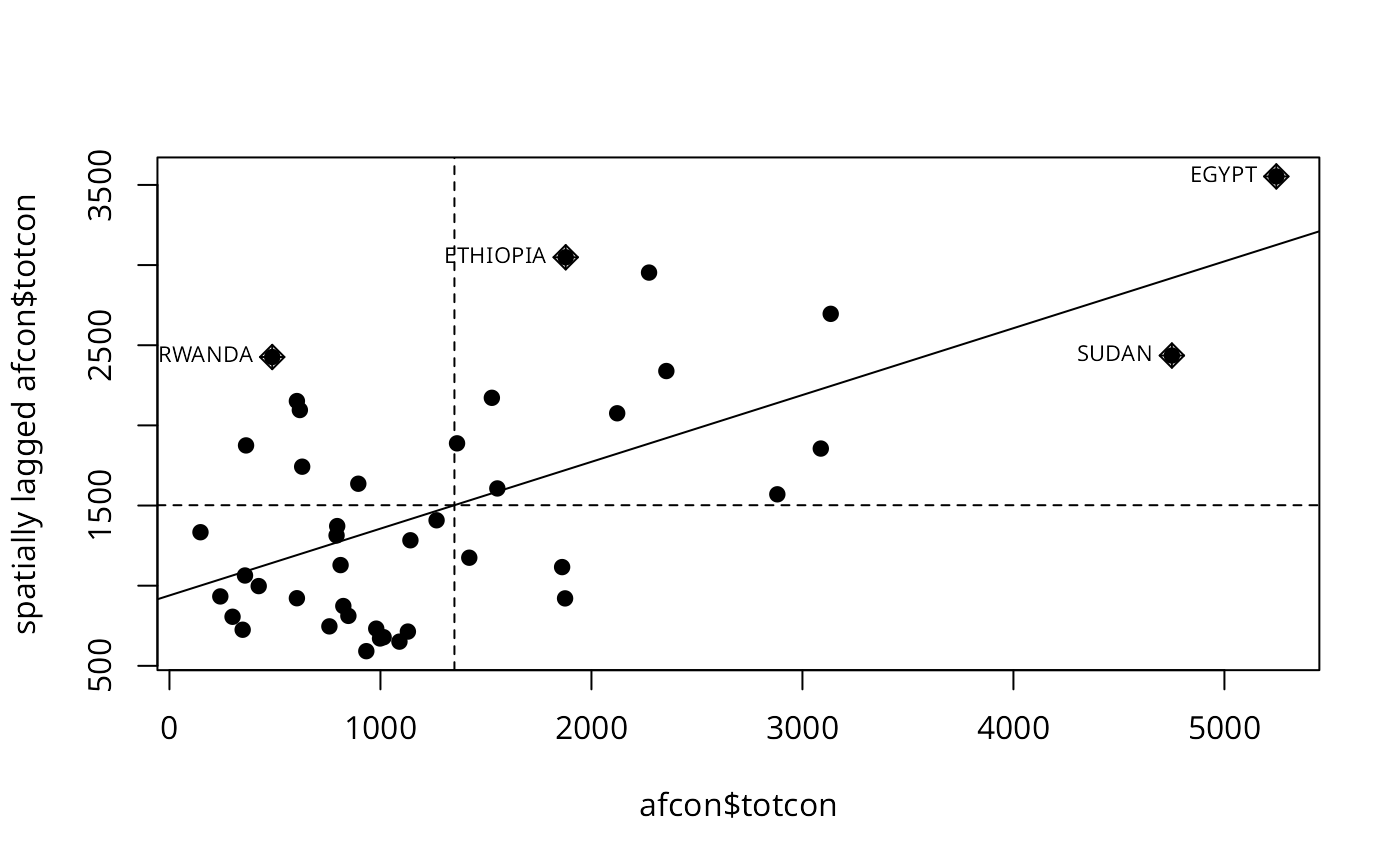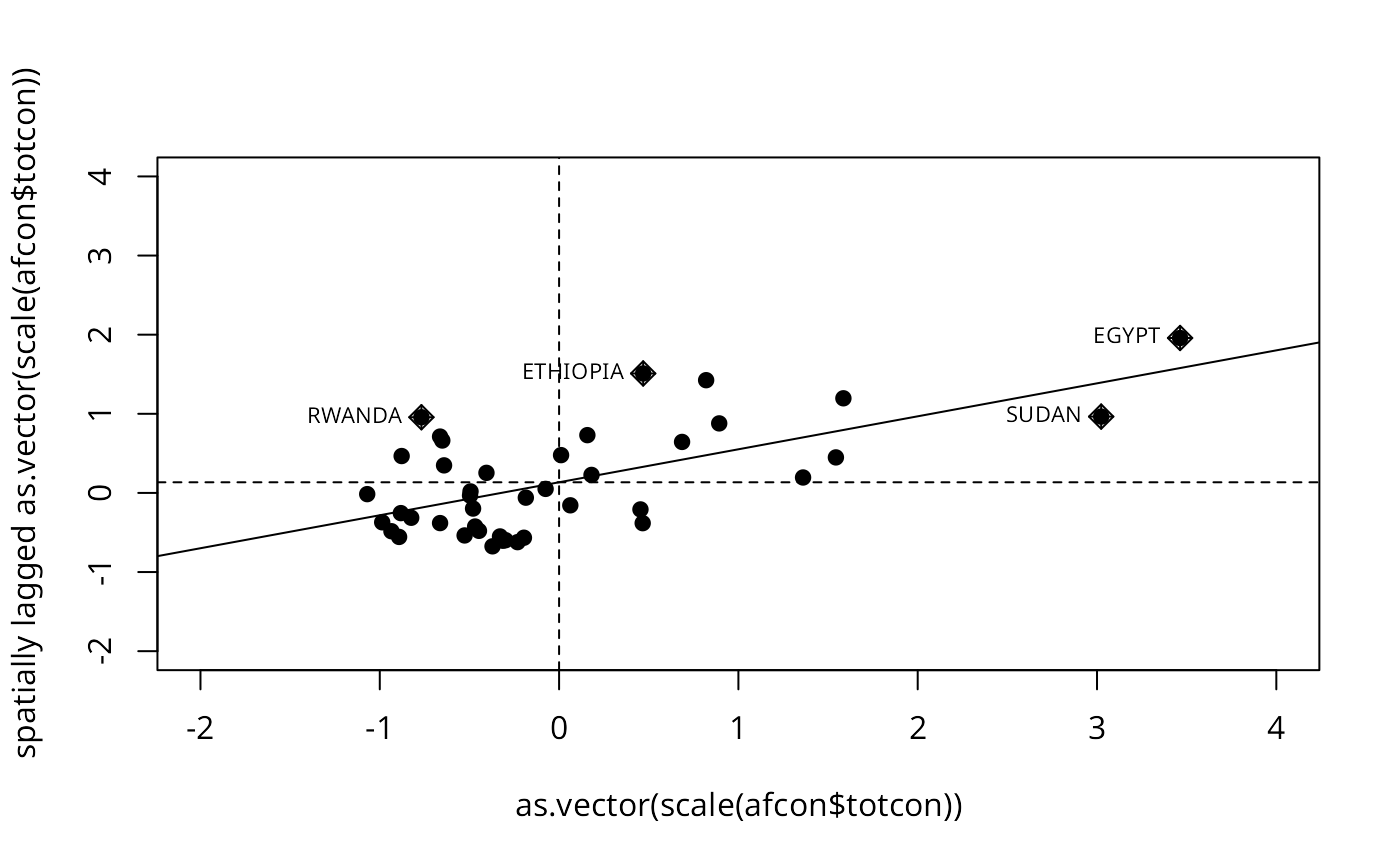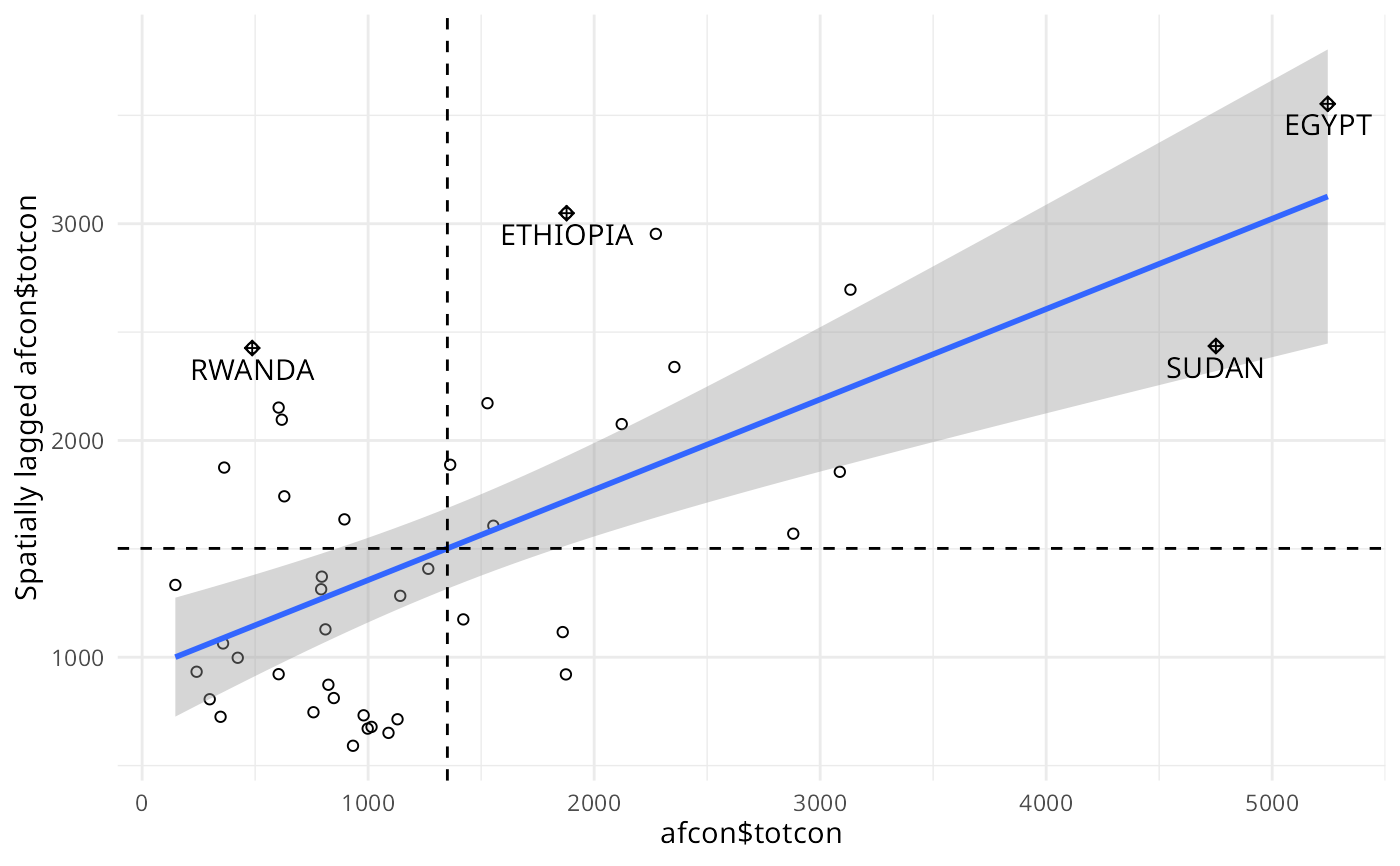A plot of spatial data against its spatially lagged values, augmented by reporting the summary of influence measures for the linear relationship between the data and the lag. If zero policy is TRUE, such observations are also marked if they occur.

moran.plot(x, listw, zero.policy=NULL, spChk=NULL, labels=NULL,
xlab=NULL, ylab=NULL, quiet=NULL, plot=TRUE, return_df=TRUE, ...)

## Arguments

x

a numeric vector the same length as the neighbours list in listw

listw

a listw object created for example by nb2listw

zero.policy

default NULL, use global option value; if TRUE assign zero to the lagged value of zones without neighbours, if FALSE assign NA

spChk

should the data vector names be checked against the spatial objects for identity integrity, TRUE, or FALSE, default NULL to use get.spChkOption()

labels

character labels for points with high influence measures, if set to FALSE, no labels are plotted for points with large influence

xlab

label for x axis

ylab

label for x axis

quiet

default NULL, use !verbose global option value; if TRUE, output of summary of influence object suppressed

plot

default TRUE, if false, plotting is suppressed

return_df

default TRUE, invisibly return a data.frame object; if FALSE invisibly return an influence measures object

...

further graphical parameters as in par(..)

## Value

The function returns a data.frame object with coordinates and influence measures if return_df is TRUE, or an influence object from influence.measures.

## Author

Roger Bivand Roger.Bivand@nhh.no

localmoran, influence.measures

## Examples

data(afcon, package="spData")
mp <- moran.plot(afcon$totcon, nb2listw(paper.nb), labels=as.character(afcon$name), pch=19)moran.plot(as.vector(scale(afcon$totcon)), nb2listw(paper.nb), labels=as.character(afcon$name), xlim=c(-2, 4), ylim=c(-2,4), pch=19)if (require(ggplot2, quietly=TRUE)) {
xname <- attr(mp, "xname")
ggplot(mp, aes(x=x, y=wx)) + geom_point(shape=1) +
geom_smooth(formula=y ~ x, method="lm") +
geom_hline(yintercept=mean(mp$wx), lty=2) + geom_vline(xintercept=mean(mp$x), lty=2) + theme_minimal() +
geom_point(data=mp[mp$is_inf,], aes(x=x, y=wx), shape=9) + geom_text(data=mp[mp$is_inf,], aes(x=x, y=wx, label=labels, vjust=1.5)) +
xlab(xname) + ylab(paste0("Spatially lagged ", xname))
}